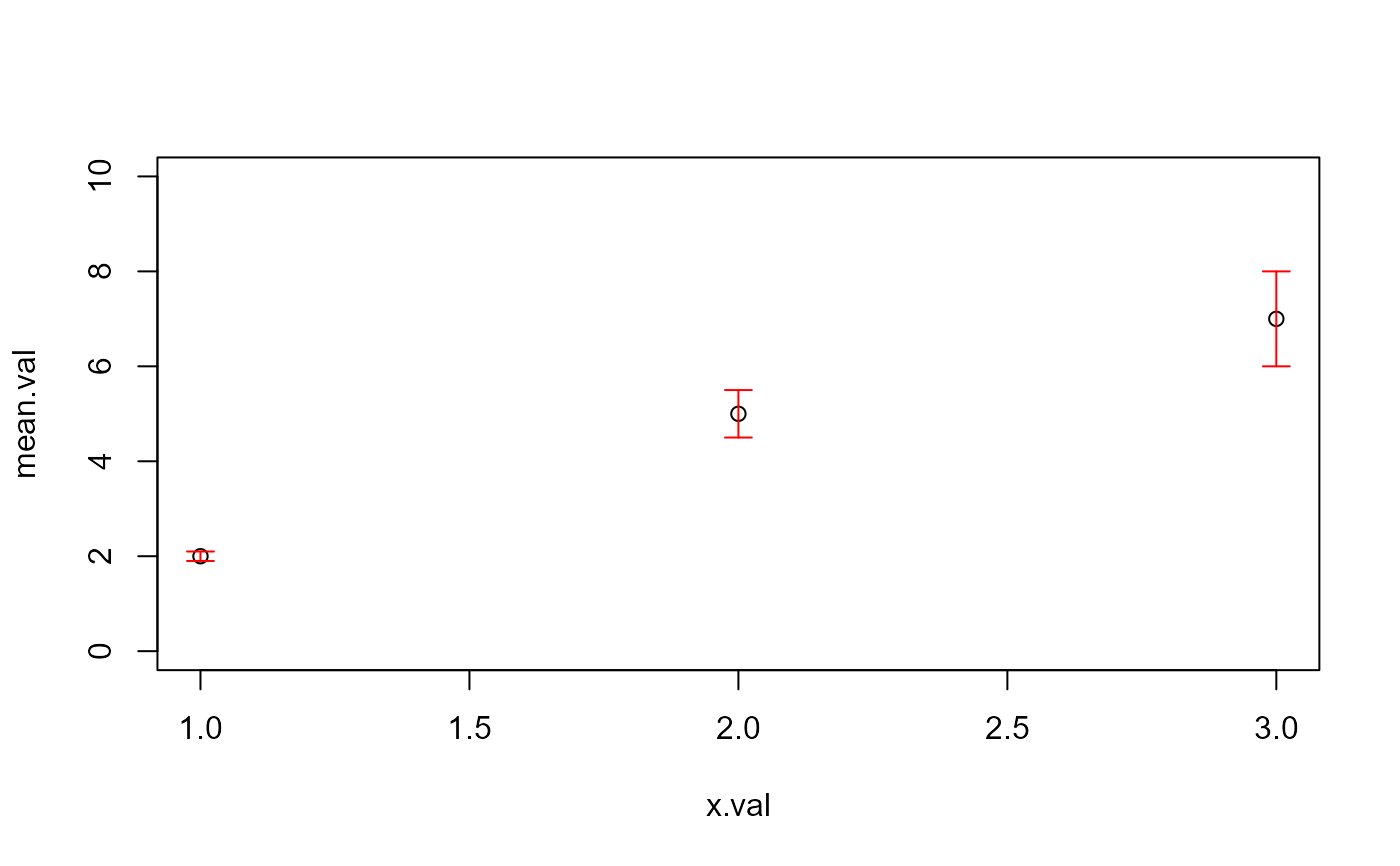Errorbars to be used with base plotting

errorbars(x, Mean, SE, col, lty = 1, length = 0.07, lwd = 1)

## Arguments

x x value mean value or y alternative standard error color of bars Line type The line type. Line types can either be specified as an integer (0=blank, 1=solid (default), 2=dashed, 3=dotted, 4=dotdash, 5=longdash, 6=twodash) or as one of the character strings "blank", "solid", "dashed", "dotted", "dotdash", "longdash", or "twodash", where "blank" uses ‘invisible lines’ (i.e., does not draw them). length of the edges of the error bar head (in inches). line width

## Value

draws lines in the form of error bars

## Note

see arrows() function

## Examples

x.val=c(1,2,3)
mean.val=c(2,5,7)
se.val=c(0.1,0.5,1)
plot(mean.val~x.val,ylim=c(0,10))errorbars(x.val,mean.val,se.val,"red")